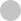#### Progress

0%#### Basic Data Structures#### Overview

•Vanilla Binary Search
•Finding Boundary

#### Sorted Array

•First Element Not Smaller Than Target 🔒
•First Occurrence
•Square Root 🔒

#### Implicitly Sorted Array#### Introduction

•Recursion Review
•DFS Fundamentals

#### Combinatorial Search

•DFS with States
•Backtracking Fundamentals
•Permutations
•Letter Combinations of Phone Number 🔒

#### Memoization

•Memoization Intro 🔒
•Word Break 🔒
•Decode Ways 🔒#### Introduction

•BFS Fundamentals

#### BFS on Tree#### Introduction

•Graph Fundamentals
•BFS on Graphs 🔒
•DFS on Graph 🔒
•BFS or DFS 🔒
•Matrix as Graph 🔒

#### BFS

•Shortest Path 🔒
•Word Ladder 🔒

#### Matrix as Graph

•Flood Fill 🔒
•Number of Islands 🔒
•Knight Minimum Moves 🔒

#### Directed Graph

•Course Schedule 🔒#### Same Direction

•Remove Duplicates 🔒
•Middle of Linked List 🔒
•Move Zeros 🔒

#### Opposite Direction

•Two Sum Sorted 🔒
•Valid Palindrome 🔒
•Trapping Rain Water 🔒

#### Prefix Sum

•Subarray Sum 🔒
•Subarray Sum Divisible by K 🔒#### Introduction

•Heap Fundamentals

#### Top K

•K Closest points 🔒
•Merge K Sorted Lists 🔒#### Binary Search#### Sequence

•House Robber 🔒
•Coin Change 🔒

#### Grid

•Number of Robot Paths 🔒
•Minimal Path Sum 🔒
•Maximal Square 🔒

#### Two Sequences

•Edit Distance 🔒
•Longest Common Subsequence 🔒

#### Game Theory

•Divisor Game 🔒#### Monotonic Stack

•Sliding Window Maximum

#### Data Structure Design

•LRU Cache 🔒# Serializing and Deserializing Binary Tree

Prereq: DFS on Tree

Given a binary tree, write a `serialize` function that converts the tree into a string and a `deserialize` function that converts a string to a binary tree. You may serialize the tree into any string representation you want as long as it can be deseralized.

## Explanation

To serialize, we can simply do a DFS and append the node value to the string. We need to encode `null` nodes too since otherwise we wouldn't be able to tell if we have reached leaf nodes when we deserialize. We use `x` here as a placeholder for the null node.

To deserialize, we split the string into tokens and consume them. For each token we create a new node using token value. When we see an `x` we know we reached the leaf and return.

Serialize

Deserialize

 `Expand 4 lines ...` `5` `5` `` self.right = right`` `6` `6` `7` `7` ``def serialize(root):`` `8` `-` `` # WRITE YOUR BRILLIANT CODE HERE`` `8` `+` `` res = []`` `9` `+` `` def dfs(root):`` `10` `+` `` if not root:`` `11` `+` `` res.append('x')`` `12` `+` `` return`` `13` `+` `` res.append(root.val)`` `14` `+` `` dfs(root.left)`` `15` `+` `` dfs(root.right)`` `16` `+` `` dfs(root)`` `17` `+` `` return ' '.join(res)`` `18` `+` `9` `19` ``def deserialize(s):`` `10` `-` `` # AND HERE`` `20` `+` `` def dfs(nodes):`` `21` `+` `` val = next(nodes)`` `22` `+` `` if val == 'x': return`` `23` `+` `` cur = Node(int(val))`` `24` `+` `` cur.left = dfs(nodes)`` `25` `+` `` cur.right = dfs(nodes)`` `26` `+` `` return cur`` `27` `+` `` # create an iterator that returns a token each time we call `next``` `28` `+` `` return dfs(iter(s.split()))`` `29` `+` `11` `30` ``if __name__ =="__main__":`` `12` `31` `` # driver code, do not modify`` `13` `32` `` def build_tree(nodes):`` `14` `33` `` val = next(nodes)`` `15` `34` `` if not val or val == 'x': return`` `Expand 14 lines ...`